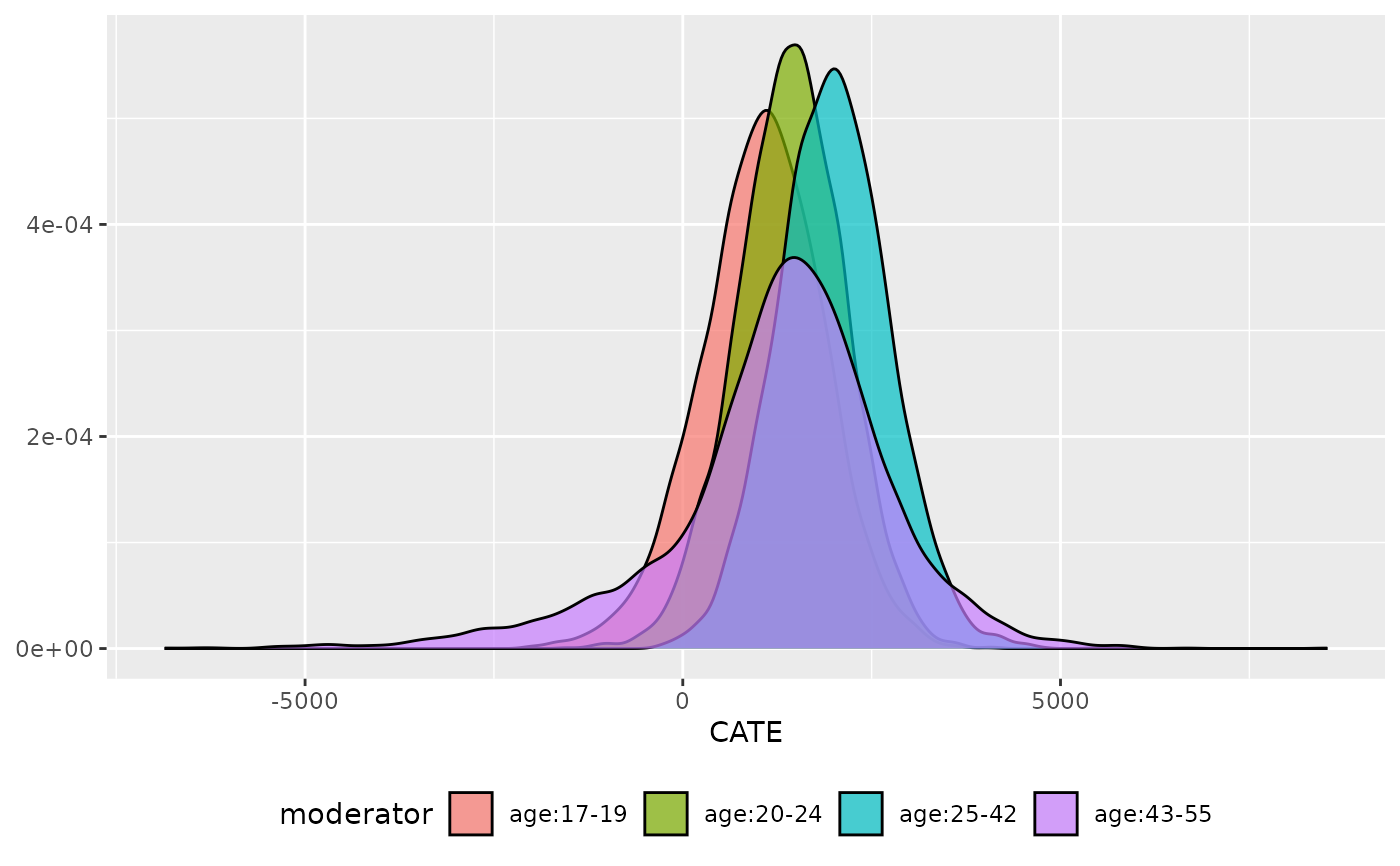Use a regression tree to optimally bin a continous variable

## Usage

plot_moderator_c_bin(
.model,
moderator,
type = c("density", "histogram", "errorbar"),
.alpha = 0.7,
facet = FALSE,
.ncol = 1,
.name = "bin"
)

## Arguments

.model

a model produced by bartCause::bartc()

moderator

the moderator as a vector

type

string to specify if you would like to plot a histogram, density or error bar plot

.alpha

transparency value [0, 1]

facet

TRUE/FALSE. Create panel plots of each moderator level?

.ncol

number of columns to use when faceting

.name

sting representing the name of the moderating variable

ggplot object

George Perrett

## Examples

# \donttest{
data(lalonde)
confounders <- c('age', 'educ', 'black', 'hisp', 'married', 'nodegr')
model_results <- bartCause::bartc(
response = lalonde[['re78']],
treatment = lalonde[['treat']],
confounders = as.matrix(lalonde[, confounders]),
estimand = 'ate',
commonSuprule = 'none'
)
#> fitting treatment model via method 'bart'
#> fitting response model via method 'bart'
plot_moderator_c_bin(model_results, lalonde\$age, .name = 'age')
#> Joining with by = join_by(splits)# }